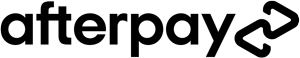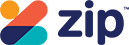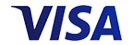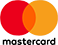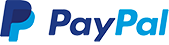Grid List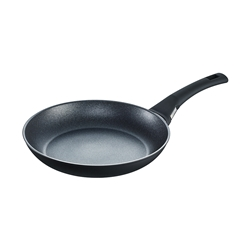WAS \$129.95 NOW \$69.95
Increase value Decrease value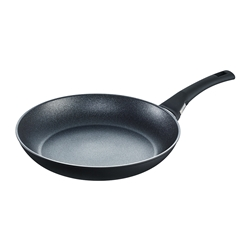\$139.95
Increase value Decrease value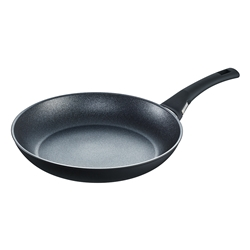WAS \$159.95 NOW \$89.95
Increase value Decrease value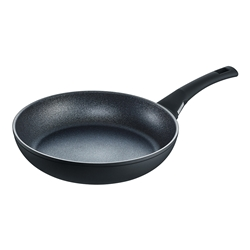WAS \$149.95 NOW \$89.95
Increase value Decrease value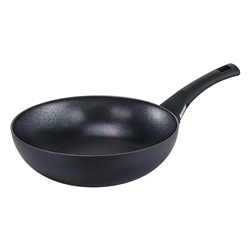WAS \$149.95 NOW \$89.95
Increase value Decrease value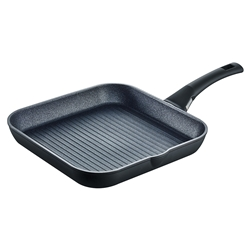WAS \$210 NOW \$125
Increase value Decrease value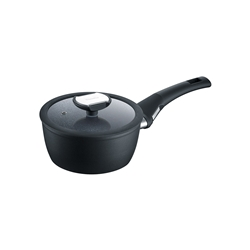WAS \$159.95 NOW \$89.95
Increase value Decrease value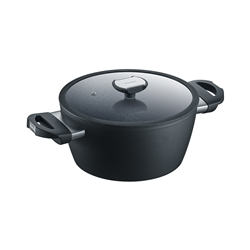WAS \$239.95 NOW \$139.95
Increase value Decrease value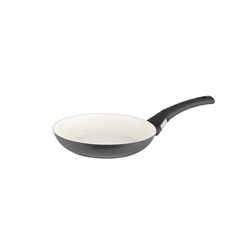\$89.95
Increase value Decrease value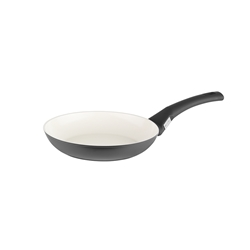WAS \$119.95 NOW \$69.95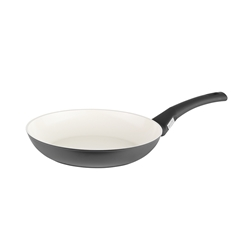\$134.95
Increase value Decrease value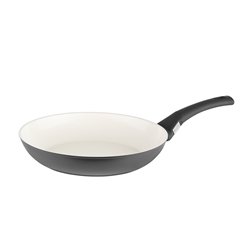\$149.95
Increase value Decrease value